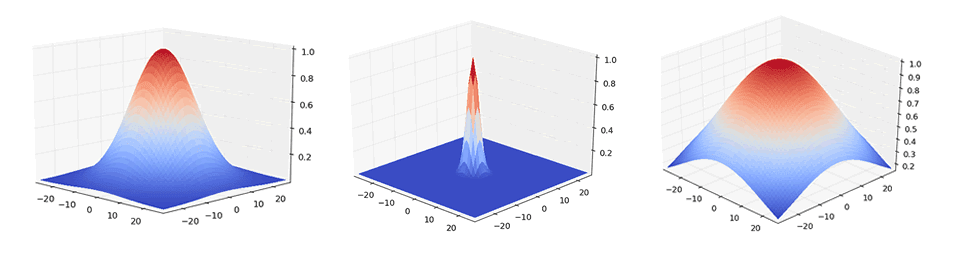## Posts

### What do Deep Networks Like to See?This is a preliminary post to explain in more detail (and hopefully in a more intuitive way) the recent findings published in the paper "What do Deep Networks Like to See?".

The quick rundown
Let's first have a look at the main findings of the paper:

We start by training an autoencoder (AE) that reconstructs images almost perfectly (it models the identity $$\mathbb{A}(x) \approx x$$ with an unsupervised MSE loss). If reconstructions are good, evaluating classification accuracy for Imagenet (using a pre-trained classifier) should yield similar results when using original images or the corresponding AE reconstructions. We found that accuracy drops quite a bit (2.83 percentage points for ResNet-50) when using reconstructions. This lower accuracy indicates that the original information contained in the image that was lost/perturbed by the AE reconstruction, was actually useful for the pre-trained classifier.We let the decoder of our AE update its parameters by passing the imag…

### How the fear of AI is the fear of science

There has been this increasing fear of AI taking over our life, mainly fed by news articles that try to convey the kind of research that is being done at the moment. Now that companies like Facebook or Google are doing major contributions to scientific research in the field of AI, it is no wonder that news outlets give those events a shot and try to bridge the gap between the sciency jargon being used in the actual papers and the main outcome, in a way that is accesible to the general public. Of course, there's also the more banal aspect of a journalist: writing an article that's catchy so people actually go and read it. Those two aspects are the ones that end up curving the authors' perspectives and we end up swimming in a sea of articles that are simply misleading. The message gets lost in translation.
This time however, we're talking about the translation between the concise, objective, peer-reviewed and expert-oriented writing that characterizes a scientific paper, …

### Math riddle: playing with infinite series

Since I haven't had that much time to work on new posts (there's also a follow up on the previous post already that I hope I can publish soon), I thought I show you a math riddle that I find quite amusing and interesting because the calculations beeing made here are completely valid (no hidden 0-divisions or tricks of that sort). I've shown this to a couple of mathematicians and they have also been surprised. So, without further ado, here it is:

$$1+2+4+8+16+...=\sum_{i=0}^{\infty}2^i = \infty$$
...but is it really $$\infty$$? Let's break it down: we can multiply the same sum by 1 without altering the result, so
$$1+2+4+8+16+... = 1\times(1+2+4+8+16+...)$$
Since $$1 = 2-1$$, we can replace it in the equation above like so:
$$1\times(1+2+4+8+16+...) = (2-1)\times(1+2+4+8+16+...)$$
$$(2-1)\times(1+2+4+8+16+...) = 2\times… ### From the sigma of a Gaussian to the size in pixelsOne of the most used filters in computer vision is the Gaussian filter. Although there are many places where you can find the formula for it, I'll support your laziness and put the 1D version of it here once more:$$G(x) =\frac{1}{\sigma\sqrt{2\pi}}e^{\frac{x^2}{2\sigma^2}}
Informally, its formula comprises two parameters which are responsible for the width $$(\sigma)$$ and the location of its tip $$(\mu)$$. The reason you can't see $$\mu$$ anywhere in the formula is because I've assumed $$\mu=0$$ which just says that the center of the Gaussian is at the center of the plane we're working. This will simplify the calculations and if you need to have your Gaussian somewhere else other than the center, you shift whatever results you get at the end to the place you actually need them.